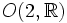Von Dyck group

This article defines a group property: a property that can be evaluated to true/false for any given group, invariant under isomorphism
View a complete list of group properties
VIEW RELATED: Group property implications | Group property non-implications |Group metaproperty satisfactions | Group metaproperty dissatisfactions | Group property satisfactions | Group property dissatisfactions

This article defines a family of groups whose members are parametrized by tuples of natural numbers. In other words, for every tuple of natural numbers, there is a unique corresponding group (upto isomorphism) in that family

Definition

Definition in terms of presentation

For natural numbers$l,m,n$, the von Dyck group$D(l,m,n)$ is defined by the following presentation:$\langle a,b,c|a^l = b^m = c^n = abc = e \rangle$

where$e$ denotes the identity element.

This is a subgroup of index two in the triangle group, but some people use the term triangle group for the von Dyck group.

Geometric description

Given natural numbers$l,m,n$, consider a triangle with sides$\pi/l, \pi/m, \pi/n$ on a suitable simply connected Riemannian surface (i.e., a suitable model for Euclidean or non-Euclidean geometry). The von Dyck group is the group generated by rotations about the vertices of the triangle by angles of$2\pi/l$,$2\pi/m$,$2\pi/n$ respectively.

The three types

Spherical von Dyck groups

The triple$(l,m,n)$ in this case satisfies:$\frac{1}{l} + \frac{1}{m} + \frac{1}{n} > 1$,

The solutions to which are$(2,3,3), (2,3,4), (2,3,5)$, and$(2,2,n)$.

This is the spherical case, with the model being the unit sphere in three-dimensional space, and the corresponding von Dyck groups are termed spherical von Dyck groups. Spherical von Dyck groups are subgroups of the special orthogonal group$SO(3,\R)$, because$SO(3,\R)$ is precisely the group of orientation-preserving isometries of the sphere. All of these turn out to be finite subgroups of$SO(3,\R)$, and these also turn out to be the only finite subgroups of$SO(3,\R)$, a fact that follows from Euler's theorem and some additional work. The finiteness can also be viewed as a consequence of the fact that the sphere is compact and simply connected. Further information: Classification of finite subgroups of SO(3,R)

Euclidean von Dyck groups

The triple$(l,m,n)$ in this case satisfies:$\frac{1}{l} + \frac{1}{m} + \frac{1}{n} = 1$,

for which the only solutions are$(4,4,2)$ and$(3,3,3)$, i.e., the right isosceles triangle and the equilateral triangle in the usual Euclidean plane.

Both of these give wallpaper groups, and neither is finite.

Hyperbolic von Dyck groups$\frac{1}{l} + \frac{1}{m} + \frac{1}{n} < 1$,

for which there are infinitely many solutions. The model for this is the hyperbolic plane.

Particular cases

Smallest parameter Middle parameter Largest parameter Common name for group Group order Symmetry object
1$n$$n$ Cyclic group$n$ Regular polygon, symmetries in$SO(2,\R)$
2 2$n$ Dihedral group$2n$ Regular polygon, symmetries in$O(2,\R)$ or in$SO(3,\R)$
2 3 3 Alternating group:A4$12$ Regular tetrahedron, symmetries in$SO(3,\R)$
2 3 4 Symmetric group:S4$24$ Cube or octahedron, symmetries in$SO(3,\R)$
2 3 5 Alternating group:A5$60$ Icosahedron or dodecahedron, symmetries in$SO(3,\R)$